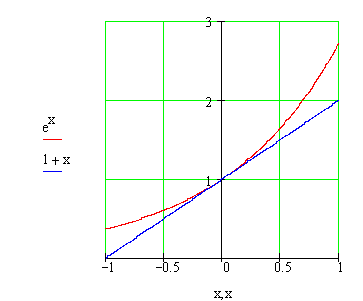Calculus on Demand at Dartmouth College Lecture 12 | Index | Lecture 14 Lecture 13## Resources

Math 3 Course Syllabus
Practice Exams

# Contents

In this lecture we develop formulas for the derivatives of exponential and logarithmic functions.

### Quick Question

What is the slope of the graph of the function ex at x = 0?### Outline

Outline for Derivatives of Exponential and Logarithm Functions

### Textbook

Derivatives of Exponential and Logarithm Functions